# 6th Grade - Coordinate Planes

## Introduction

• The coordinate plane is a 2-D surface divided into 4 quadrants by two intersecting lines called the X-axis and Y- axis.
• The point where the two axes intersect is called the origin. It is represented by coordinates (0,0).

## The X-axis and The Y-axis

• The X- axis is the horizontal line (just like the integers number line), where all the numbers to the right of the "0" are positive and to the left of the 0 are negative.
• The Y-axis is the vertical line (just like the vertical integers number line), where all the numbers above 0 are positive and below 0 are negative.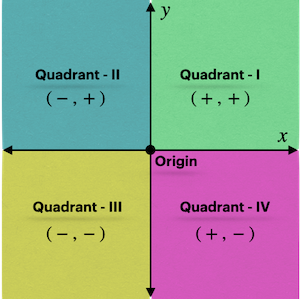• Quadrant I - Located in the upper right of the coordinate plane where the X-axis and Y-axis both have positive numbers.
• Quadrant II - Located in the upper left of the coordinate plane where the X-axis has negative and the Y-axis has positive numbers.
• Quadrant III - Located in the lower left of the coordinate plane where the X-axis and Y-axis both have negative numbers.
• Quadrant IV - Located in the lower right of the coordinate plane where the X-axis has positive numbers and the Y-axis has negative numbers.

## The Coordinates

• The coordinates are the set of ordered pairs of numbers written within the parentheses.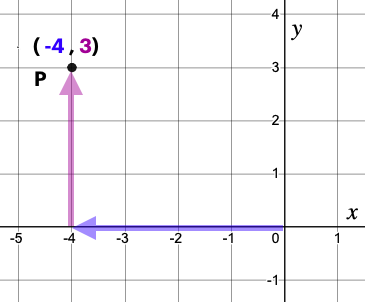• The first number of the ordered pair shows the distance of the point from the origin on the X-axis.
• The second number of the ordered pair shows the distance of the point from the origin on the Y- axis.
• The coordinates represent the exact position of a point on the coordinate plane.

## Finding Distance between Two Points

• To find the distance between any two points lying on the horizontal line (x-axis) or vertical line (y-axis):
• Plot the given points on a coordinate plane (to identify whether the points lie on the horizontal line or vertical line).
• Count the number of units between the two given points.
• For example, the distance between the two points M(2, 6) and N(2, 3), as shown on the coordinate plane, is 3 units.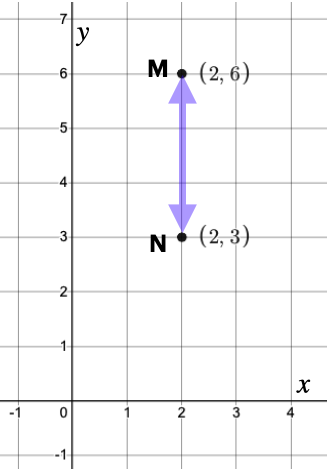• Note: The distance between any two points on a coordinate plane can never be negative.

• To find the perimeter of the quadrilateral on the coordinate plane:

1. Find the coordinates of the given points.

2. Connect the points so that any two lines meet only at the points (corners).

3. Find the distance between all the points connected by a straight line.

4. Add up the distance between the points.

## Solved Examples

Example 1: Write the coordinates of the point P shown in the graph below.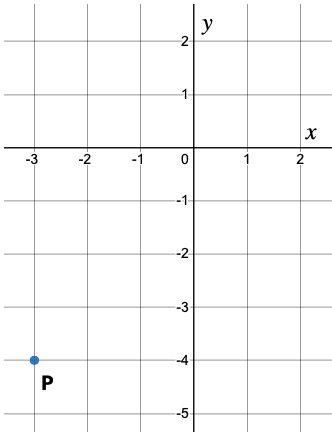Solution: Point P lies in the third quadrant. Looking at the graph, we can conclude that .

Example 2: Find the distance between the points M and N as shown in the graph below.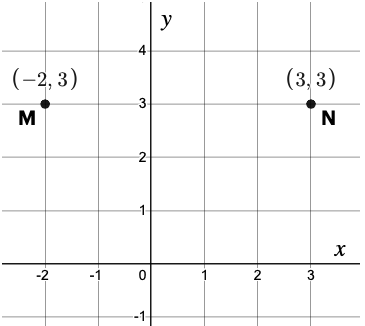Solution: The given points lie on a horizontal line. The coordinates for M are (-2, 3), and so it is |-2| units left to the y-axis. Similarly, the coordinates for point N are (3, 3), and it is |3| units right to the y-axis.

Thus the distance between the points M and N is

|-2| + |3| = 2 + 3 = 5 units as shown in the graph below.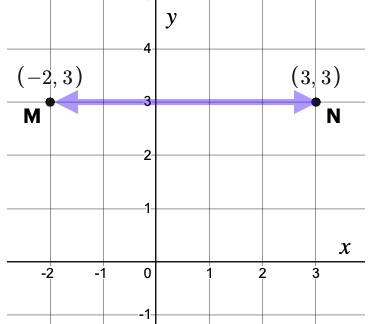Example 3:Which point represents the point (-3, 2)?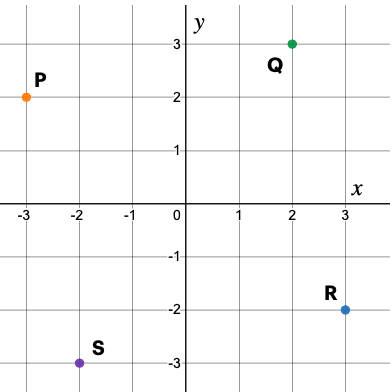Solution: The point (-3, 2) must lie in the second quadrant. Hence, point P represents the given point.

Example 4: Find the perimeter of the rectangle ABCD shown below.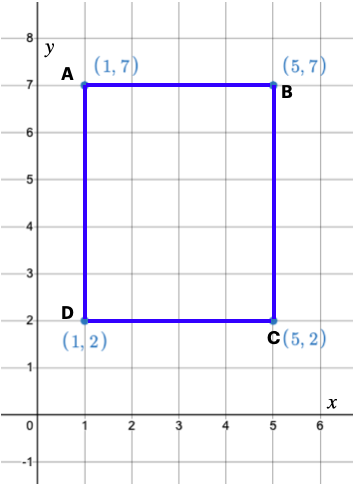Solution: Perimeter of a rectangle is the sum of the length of all its sides.

AB = 5 - 1 = 4 (horizontal line)

BC = 7 - 2 = 5 (vertical line)

CD = 5 - 1 = 4 (horizontal line)

DA = 7 - 2 = 5 (vertical line)

Perimeter of rectangle ABCD = AB + BC + CD + DA

= 4 + 5 + 4 + 5 = 18 units

## Cheat Sheet

• Naming quadrants go anti-clock-wise. See the above image.
• The coordinates of a point that lies in quadrant I are positive.
• The coordinates of a point that lies in quadrant III are negative.
• The distance between any two points lying on the horizontal line (x-axis) or vertical line (y-axis) can be found by simply counting the units between the two points.

## Blunder Area

• While writing ordered pairs of a point, the X - coordinate always goes first.
• The coordinates of any point are always written within the parentheses.

## Distance Formula (not for Grade 6)

• To find the distance between any two points on the coordinate plane as shown in the figure below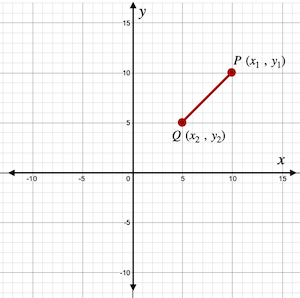• Find the coordinates of both points. Here  and .
• Use the distance formula:

$\text{distance}=\sqrt{{\left(∆x\right)}^{2}+{\left(∆y\right)}^{2}}$ ,where $△x=$change in abscissae and $△y=$change in ordinates.

$\text{distance}=\sqrt{{\left({x}_{2}-{x}_{1}\right)}^{2}+{\left({y}_{2}-{y}_{1}\right)}^{2}}=\sqrt{{\left({x}_{1}-{x}_{2}\right)}^{2}+{\left({y}_{1}-{y}_{2}\right)}^{2}}$